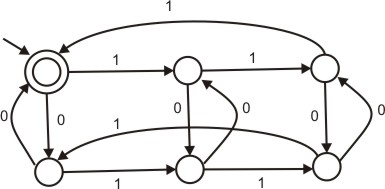Related Articles
GATE | GATE-CS-2004 | Question 86
• Last Updated : 26 Sep, 2014

The following finite state machine accepts all those binary strings in which the number of 1’s and 0’s are respectively.(A) divisible by 3 and 2
(B) odd and even
(C) even and odd
(D) divisible by 2 and 3

Answer: (A)

Explanation:

Option (B) is eliminated because string 100 contains odd number of 1s and even number of 0s but is not accepted by the DFA.
Option(C) is eliminated because string 011 contains even number of 1s and odd number of 0s but is not accepted by the DFA.
Option (D) is eliminated because string 11000 has number of 1s divisible by 2 and number of 0s divisible by 3 but still not accepted by the DFA.
Option (A) accepts all strings with number of 1s divisible by 3 and number of 0s divisible by 2.

Extra note: In any case where (no of 1s) MOD N= some integer k and (no of 0s) MOD M= some integer q the number of states in the DFA will be equal to N*M.
(The product could be taken for all input alphabets.)
E.g.: if we say no. of ones is even and no. of 0s is odd (we check if (no. of 1s) MOD 2=0 and (no. of 0s) MOD 2=0) so no. of states in the DFA=2*2=4.
Hence option (B) and (C) can directly be eliminated as the DFA has 6 states and we can look only at the remaining two options.

This solution is contributed by Yashika Arora .My Personal Notes arrow_drop_up